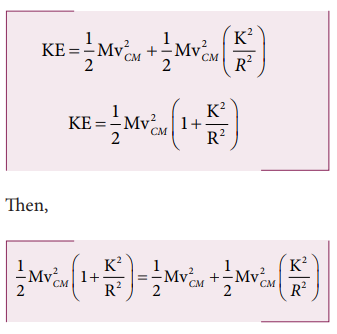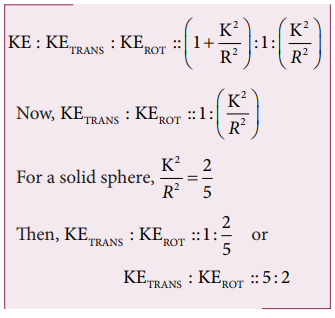Home | | Physics 11th std | Solved Example Problems for Kinetic Energy in Pure Rolling(Rolling Motion)

# Solved Example Problems for Kinetic Energy in Pure Rolling(Rolling Motion)

Physics : Motion of System of Particles and Rigid Bodies : Rolling Motion : Kinetic Energy in Pure Rolling

Solved Example Problems for Kinetic Energy in Pure Rolling(Rolling Motion)

### Example 5.22

A solid sphere is undergoing pure rolling. What is the ratio of its translational kinetic energy to rotational kinetic energy?

### Solution

The expression for total kinetic energy in pure rolling is,For any object the total kinetic energy as per equation 5.58 and 5.59 is,The above equation suggests that in pure rolling the ratio of total kinetic energy, translational kinetic energy and rotational kinetic energy is given as,Study Material, Lecturing Notes, Assignment, Reference, Wiki description explanation, brief detail
11th Physics : UNIT 5 : Motion of System of Particles and Rigid Bodies : Solved Example Problems for Kinetic Energy in Pure Rolling(Rolling Motion) |